Next: 3.2.8 Shockley-Read-Hall Generation/Recombination Up: 3.2 Material Models Previous: 3.2.6 Saturation Velocity

## 3.2.7 Energy Relaxation Times

For simulation of HEMTs in  constant energy relaxation times were used. Table 3.20 compiles data for different materials which were used with the extended Hänsch mobility model, as in . Data for In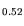Al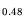As and In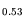Ga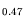As are added in this work. Constant energy relaxation times do not allow for the modeling of effects such as static velocity overshoot in combination with the mobility model used in (3.46) which was originally developed for Si.

Table 3.20: Constant energy relaxation times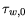fitted for III-V HEMTs.
 Material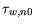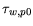[ps] [ps] Al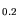Ga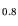As 0.1 0.1 InGaAs 0.17 0.1 InGaAs 0.17 0.1 InAlAs 0.1 0.1

A constant energy relaxation time is an approximation, since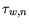and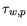are, in a first order approach, functions of the average energy in III-V semiconductors. Using a constant, the modeling combining (3.49) and Table 3.20 causes numerical problems for the evaluation of the mobility. For carrier cooling, i.e., for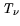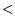for arbitrarythe result is a complex number for the mobility in (3.49), which means, that a lower bound for=must be arbitrarily introduced.

Several approaches have undertaken to model the dependence of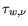using splines in  or analytical functions in . The model usually includes the dependence on carrier temperature, lattice temperature, and material composition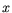for given doping concentration. Thus, the energy relaxation timeis considered a single valued function of the carrier temperature. The error introduced by this assumption in relation to the hydrodynamic implementation is discussed by Grasser et al. in . For homogeneous bulk binary semiconductors in  the following relation is suggested: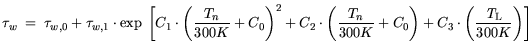(3.49)

For the ternary alloys relation (3.53) is used: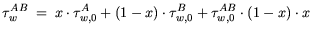(3.50)

and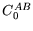is modeled according to: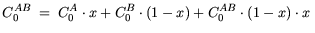(3.51)

In Table 3.21 and Table 3.22 values are given that were found from fitting the extraction of bulk MC data. For holes constant values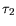=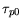are used.

Table 3.21: Energy relaxation times for electrons and holes in III-V semiconductors.
 Material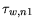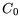C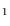C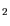C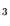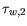[ps] [ps] [ps] GaAs 0.48 0.025 0 -0.053 1.322 0 0.1 AlAs 0.17 0.025 61 -0.053 0.853 0.5 0.1 InAs 0.08 0.025 3 -0.053 0.853 0.5 0.1

Table 3.22: Composition dependence of the III-V ternary semiconductors.
 Material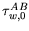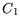CC[ps] [ps] [ps] AlGaAs -0.35 0.025 -61 -0.053 0.853 0.5 0.1 InGaAs 1.8 0.025 -34 -0.053 0.853 0.5 0.1 InAlAs -0.4 0.025 -34 -0.053 0.853 0.5 0.1

Given the specific hydrodynamic transport model and the mobility model used in this work, it is found, that the parameters from  do neither allow to obtain the correct electron temperatures nor the correct velocities in the model obtained by two-dimensional MC simulations for the specific HEMT device situation. The consideration of the hydrodynamic model discussed by Grasser et al. in [107,108] name the finite number of moments considered responsible for the mismatch. Additionally, the following physical arguments shall be given: Comparing the values of Table 3.20 and Table 3.21, the constant values obtained in previous work are smaller than the minimum values of the energy relaxation times extracted from bulk MC simulations. One reason for this is the occurrence of heterointerfaces, which are naturally most relevant for HEMTs. Comparing to silicon, in  the electron relaxation time is extracted to be of the order of= 0.1 ps from a MC study including the quantization at the SiOinterface. This value is significantly smaller than the bulk value in Si of about= 0.3 ps . Additionally, for HEMT simulations especially for the channel the existence of Real Space Transfer (RST) modifies the high field transport for the analytical model and has to be discussed considering the hydrodynamic interface model used. Ultimate verification of carrier temperatures and velocities is achieved by one- and two-dimensional device MC simulations which include the effect of RST.

For this work energy dependent relaxation times have been used. For the energy dependence the above considerations lead to a consistent scaling of the energy relaxation functions by the same factor and is considered fitting function within a consistent model, as discussed in Section 3.6. Thus, the disagreement provided in Table 3.20 and Table 3.21 can be resolved. For the scaling of the functions (3.52) and the parameters, a down scaling by the factor of 8 of the resulting energy relation times using Table 3.21 was found to give generally good agreement with MC simulations for all materials. If investigations with single bias were performed constant ERTs (see Table 3.20) can also be taken using the saturation velocity as a fit parameter. However, as shown in Section 3.6, for any multi bias investigations energy dependent ERT should be used.Next: 3.2.8 Shockley-Read-Hall Generation/Recombination Up: 3.2 Material Models Previous: 3.2.6 Saturation Velocity
Quay
2001-12-21